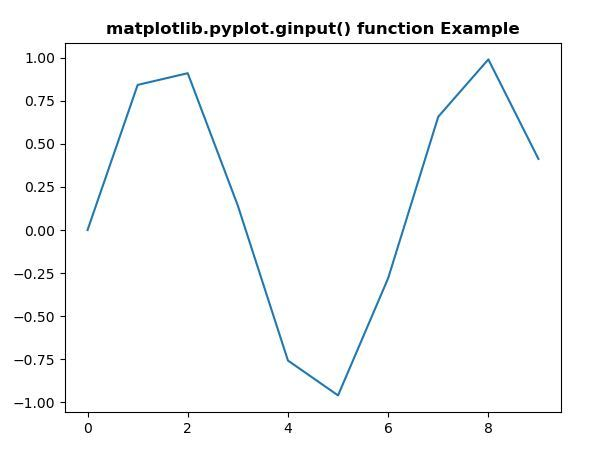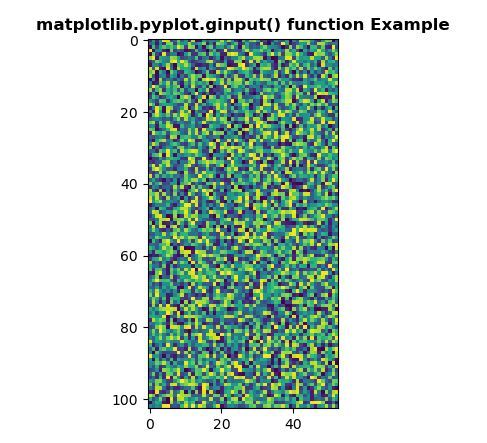Related Articles

# Matplotlib.pyplot.ginput() in Python

• Last Updated : 30 Apr, 2020

Matplotlib is a library in Python and it is numerical – mathematical extension for NumPy library. Pyplot is a state-based interface to a Matplotlib module which provides a MATLAB-like interface. There are various plots which can be used in Pyplot are Line Plot, Contour, Histogram, Scatter, 3D Plot, etc.

## matplotlib.pyplot.table() Function

The ginput() method pyplot module of matplotlib library is used to block call to interact with a figure.

Syntax: matplotlib.pyplot.ginput(n=1, timeout=30, show_clicks=True, mouse_add=1, mouse_pop=3, mouse_stop=2)

Parameters: This method accept the following parameters that are described below:

• n : This parameter is the number of mouse clicks to accumulate.
• timeout : This parameter is the number of seconds to wait before timing out.
• show_clicks : This parameter is used to show a red cross at the location of each click.
• mouse_add : This parameter is the Mouse button used to add points.
• mouse_pop : This parameter is the Mouse button used to remove the most recently added point.
• mouse_stop : This parameter is the Mouse button used to stop input.

Returns: This method return the list of the clicked (x, y) coordinates.

Below examples illustrate the matplotlib.pyplot.ginput() function in matplotlib.pyplot:

Example 1:

 `# Implementation of matplotlib function``import` `matplotlib.pyplot as plt``import` `numpy as np``t ``=` `np.arange(``10``)``  ` ` ` `plt.plot(t, np.sin(t))``    ` `plt.title('matplotlib.pyplot.ginput()\`` ``function Example', fontweight ``=``"bold"``)``  ` `print``(``"After 3 clicks :"``)``x ``=` `plt.ginput(``3``)``print``(x)``  ` `plt.show()`

Output:```After 3 clicks :
[(4.460080645161289, 0.5915838985273842),
(4.460080645161289, 0.5915838985273842),
(4.460080645161289, 0.5915838985273842)]
```

Example 2:

 `# Implementation of matplotlib function``import` `matplotlib.pyplot as plt``import` `numpy as np``  ` ` ` `np.random.seed(``10``*``*``7``)``    ` `x1 ``=` `np.random.rand(``103``, ``53``) ``    ` `plt.title('matplotlib.pyplot.ginput() function\`` ``Example', fontweight ``=``"bold"``)``  ` `print``(``"After 2 clicks :"``)``plt.imshow(x1)``x ``=` `plt.ginput(``2``) ``print``(x)``  ` `plt.show()`

Output:```After 2 clicks :
[(8.443181818181813, 38.90530303030302),
(8.443181818181813, 38.90530303030302)]
```

Attention geek! Strengthen your foundations with the Python Programming Foundation Course and learn the basics.

To begin with, your interview preparations Enhance your Data Structures concepts with the Python DS Course. And to begin with your Machine Learning Journey, join the Machine Learning – Basic Level Course

My Personal Notes arrow_drop_up